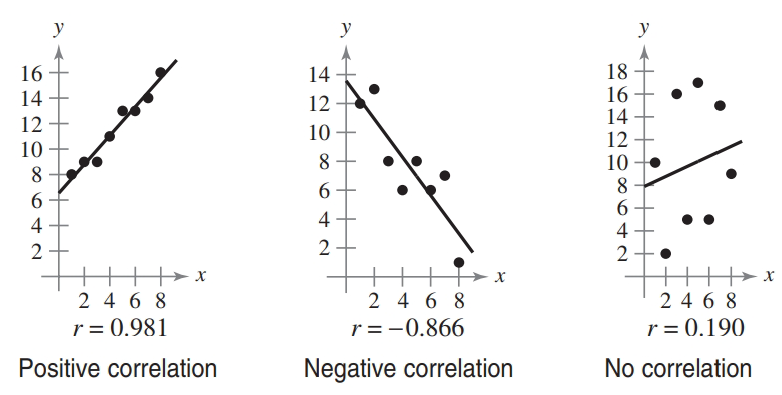Chapter 7.7, Problem 21E### Calculus: An Applied Approach (Min...

10th Edition
Ron Larson
ISBN: 9781305860919

#### Solutions

Chapter
Section### Calculus: An Applied Approach (Min...

10th Edition
Ron Larson
ISBN: 9781305860919
Textbook Problem
12 views

# Determining Correlation In Exercises 19-24, plot the points and determine whether the data have positive, negative, or no linear correlation (see figures below). Then use a graphing utility to find the value of r and confirm your result. The number r is called the correlation coefficient. It is a measure of how well the model fits the data. Correlation coefficients vary between -1 and 1, and the closer |r| is to 1, the better the model.(1,3), (2, 6), (3, 2), (4, 3), (5, 9), (6, 1)To determine

To graph: The points (1,3),(2,6),(3,2),(4,3),(5,9),(6,1) and determine whether these points have positive, negative or no linear correlation. Also determine the correlation coefficient using graphing utility.

Explanation

Given Information:

The provided points are (1,3),(2,6),(3,2),(4,3),(5,9),(6,1).

Graph:

Consider the provided points,

(1,3),(2,6),(3,2),(4,3),(5,9),(6,1)

Label these points on coordinate axis and connect them with a straight line as shown below,

### Still sussing out bartleby?

Check out a sample textbook solution.

See a sample solution

#### The Solution to Your Study Problems

Bartleby provides explanations to thousands of textbook problems written by our experts, many with advanced degrees!

Get Started

#### Find more solutions based on key concepts